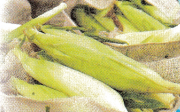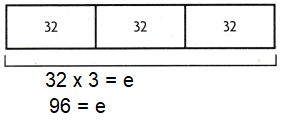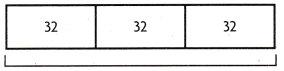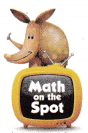# Texas Go Math Grade 4 Lesson 11.4 Answer Key Multi-Step Division Problems

Refer to our Texas Go Math Grade 4 Answer Key Pdf to score good marks in the exams. Test yourself by practicing the problems from Texas Go Math Grade 4 Lesson 11.4 Answer Key Multi-Step Division Problems.

## Texas Go Math Grade 4 Lesson 11.4 Answer Key Multi-Step Division Problems

Essential Question

How can you use the strategy draw a diagram to solve multi-step division problems?
In solving multi-step division problems, the total to be found first,
Then the total should be divided in to the required equal parts.

Unlock the ProblemLucia and her dad will prepare corn for a community picnic. There are 3 bags of corn. Each bag holds 32 ears of corn. When the corn is cooked, they want to divide the corn equally among B serving plates. How many ears of corn should they put on each of 8 serving plates?
12 ears of corn.
Explanation:
There are 3 bags of corn.
Each bag holds 32 ears of corn.
3 x 32 = 96
When the corn is cooked, they want to divide the corn equally among 8 serving plates.
Total ears of corn should they put on each of 8 serving plates
96÷8 = 12

What do I need to find?
I need to find the number of ________________ that will go on each plate.
Ear corns

What information am I given?
______________ bags with ______________ ears in each bag. The total ears arc divided equally into ______________ groups.
3 bags with 32 ears in each bag.
8 groups.

Plan

What is my plan or strategy?

I will make a strip diagram for each step and use equations. Then I will ______________ to find the total and ___________ to find the number for each plate.I will multiply 32 x3  to find the total and divide 96 to find the number for each plate.

Solve

I can draw strip diagrams and use equations and then decide how to find how many ears of corn should go on a plate.

First, I will find the total number of ears of corn.32 × __________ = e
___________ = e
Then I will find how many ears of corn should go on each plate.96 ÷ __________ = c
___________ = c96 ÷ 8 = c
12 = c

Question 1.
How many ears of corn should go on each plate?
12 ears of corn

Question 2.
32 × 3 = e
96 = e
96 ÷ 8 = c
12 = c
Explanation:
Division is opposite to multiplication and multiplication is opposite to division vice-versa.

Try Another Problem

There are 8 dinner rolls in a package. How many packages will be needed to feed 64 people if each person has 2 dinner rolls?What do I need to find?
Total packages will be needed to feed 64 people if each person has 2 dinner rolls.

What information am I given?
Each package contains 8 dinner rolls.
Total number of people to feed 64.

Plan

What is my plan or strategy?
I will make a strip diagram for each step and use equations.

Solve
64 x 2 = 128 dinner rolls required
128 ÷ 8 = p
16 = p
Question 3.
How many packages of rolls will he needed?
16 packages of rolls needed for 64 people for dinner

Question 4.
Strip Drawing helps to break the whole into small parts to slove.Math Talk

Mathematical Processes
Describe another method you could have used to solve the problem.
Equation method
Explanation:
64 x 2 =128
128 ÷ 8 = 16

Share and Show

Question 1.
A firehouse pantry has 52 cans of vegetables and 74 cans of soup. Each shelf holds 9 cans. What is the the least number of shelves needed for all the cans?

First, draw a strip diagram for the total number of cans.
Next, add to find the total number of cans.
Then, draw a strip diagram to show the number of shelves needed.
Finally, divide to find the number of shelves needed.So, _________ Shelves are needed to hold all of the cans.
14 shelves are needed to hold all of the cans.
Explanation:
A firehouse pantry has 52 cans of vegetables and 74 cans of soup.
Each shelf holds 9 cans.
The least number of shelves needed for all the cans
(52 + 74) ÷ 9 = n
126 ÷ 9 = n
14 = n

Question 2.
H.O.T. Multi-Step What if 18 cans fit on a shelf? What is the least number of shelves needed? Describe how your answer would be different.
7 shelves are needed to hold all of the cans
Explanation:
A firehouse pantry has 52 cans of vegetables and 74 cans of soup.
Each shelf holds 18 cans.
The least number of shelves needed for all the cans
(52 + 74) ÷ 18 = n
126 ÷ 18 = n
7 = n

Problem-Solving

Question 3.H.O.T. Multi-Step Ms. Johnson bought 6 bags of balloons. Each hag has 25 balloons. She fills all the balloons and puts 5 balloons in each bunch. How many bunches can she make?
30 bunches.
Explanation:
Ms. Johnson bought 6 bags of balloons.
Each bag has 25 balloons.
She fills all the balloons and puts 5 balloons in each bunch.
Total bunches she can make
(6 x 25) ÷ 5 = n
150 ÷ 5 = n
30 = n

Question 4.
Basketball jerseys are shipped in packages of 6. How many packages of jerseys are needed for 12 players if each player gets 4 jerseys?
(A) 3 packages
(B) 2 packages
(C) 8 packages
(D) 48 packages
Option(B)
Explanation:
Basketball jerseys are shipped in packages of 6,
if each player gets 4 jerseys.
Total packages of jerseys are needed for 12 players, if each player gets 4 jerseys
(6 x 4) ÷ 12 = n
24 ÷ 12 = n
2 = n

Question 5.
Robin and her grandmother bake muffins. Each batch of hatter makes 36 mini muffins. They make 4 batches. They divide the muffins equally into bags of 3 muffins for a bake sale. How many bags of 3 muffins do they have?
(A) 9 bags
(B) 48 bags
(C) 12 bags
(D) 3 bags
Option(B)
Explanation:
Robin and her grandmother bake muffins.
Each batch of batter makes 36 mini muffins.
They make 4 batches.
They divide the muffins equally into bags of 3 muffins for a bake sale.
Total bags of 3 muffins they have
(36 x 4) ÷ 3 = n
144 ÷ 3 = n
48 = n

Question 6.
Multi-Step The fourth graders at Sunshine School go to the Museum of Nature and Science. The school pays $671 for the trip. The adult tickets are$10 each and the student tickets are $7 each. There are 9 adults going on the trip. How many students go on the trip? (A) 74 students (B) 86 students (C) 95 students (D) 83 students Answer: Option(D) Explanation: The school pays$671 for the trip.
The adult tickets are $10 each and the student tickets are$7 each.
There are 9 adults going on the trip.
Total students go on the trip
671 – (10 x 9) ÷ 7  = n
90 – 671 ÷ 7= n
581 ÷ 7 = n
83 = n

TEXAS Test Prep

Question 7.
Ben collected 43 cans and some bottles. He received 5 for each can or bottle. If Ben received a total of $4.95, how many bottles did he collect? (A) 56 (B) 99 (C) 560 (D) 990 Answer: Option(A) Explanation: Ben collected 43 cans and some bottles. He received 5 for each can or bottle. If Ben received a total of$4.95,
Number of bottles he collect
(43 + x) x 0.0 5 = 2.15 + 0.05x
4.95 = 2.15 + 0.05x
4.95 – 2.15 = 0.05x
2.80 = 0.05x
2.80 ÷ 0.05 = x
x = 56

#### Texas Go Math Grade 4 Lesson 11.4 Homework and Practice Answer Key

Problem Solving

Question 1.
Marco bought 2 bottles of juice. Each bottle is 48 ounces. How many 8-ounce glasses of juice can Marco pour from the two bottles?
12 glasses.
Explanation:
48 + 48 = 96 ounces
96 ÷ 8 = 12 glasses

a. Draw a strip diagram for the number of ounces of juice in the two bottles.b. Write an equation to find the total number of ounces of juice in the two bottles.
n = 2 x 48 = 96 ounces

c. Draw a strip diagram to show the number of 8-ounce glasses of juice that can be poured.d. Write an equation to find the number of glasses of juice.

Marco can pour ________ glasses of juice.
12 glasses.
Explanation:
x = 96 ÷ 8 = 12 glasses
Marco can pour 12 glasses of juice

Question 2.
Describe another method you could have used to solve the problem.
Algebraic equation method
n=48 x 2 = 96
x = 96 ÷ 8 = 12

Question 3.
What if Marco poured 10-ounce glasses of juice? What is the greatest number of full glasses of juice he could have poured? Explain.
x = 96 ÷ 10 = 9.6

Lesson Check

Question 4.
Multi-Step Orlando has a bag of 37 apples and a bag of 29 apples. He can hake 6 apples in a pan. How many pans of apples can Orlando make?
(A) 66
(B) 33
(C) 11
(D) 22
Option(C)
Explanation:
Orlando has a bag of 37 apples and a bag of 29 apples.
He can bake 6 apples in a pan.
Total pans of apples Orlando can make
(37 + 29) ÷ 6 = n
66 ÷ 6 = n
11 = n

Question 5.
Multi-Step Anna has 5 bunches of flowers with 12 flowers in each bunch. She has 4 bunches of flowers with 10 flowers in each bunch. How many vases can Anna fill if she puts 10 flowers in each vase?
(A) 10
(B) 100
(C) 9
(D) 15
Option(A)
Explanation:
Anna has 5 bunches of flowers with 12 flowers in each bunch.
She has 4 bunches of flowers with 10 flowers in each bunch.
Total vases Anna can fill if she puts 10 flowers in each vase
(5 x 12) + (4 x 10) ÷ 10 = n
60 + 40 ÷ 10 = n
100 ÷ 10 = n
10 = n

Question 6.
Multi-Step Five friends are going to share the cost of two gifts. One gift costs $39 and the other gift costs$26. What is each person’s share of the cost?
(A) $7 (B)$15
(C) $18 (D)$13
Option(D)
Explanation:
Five friends are going to share the cost of two gifts.
One gift costs $39 and the other gift costs$26.
Each person’s share of the cost
39 + 26 ÷ 5 = n
65 ÷ 5 = n
13 = n

Question 7.
Multi-Step There are 14 chairs in each of 6 rows. There are 18 chairs in each of 4 rows. How many rows of 13 chairs can be made from all of the chairs?
(A) 13
(B) 12
(C) 14
(D) 18
Option(B)
Explanation:
There are 14 chairs in each of 6 rows.
There are 18 chairs in each of 4 rows.
Total rows of 13 chairs can be made from all of the chairs
(14 x 6) + (18 x 4) ÷ 13 = n
(84 + 72 ) ÷ 13 = n
156 ÷ 13 = n
12 = n

Question 8.
Multi-Step Justin collected 26 shells, Amy collected 31 shells. Jose collected 21 shells, If they share all of the shells equally, how many shells will each person get?
(A) 24
(B) 78
(C) 26
(D) 19
Option(C)
Explanation:
Justin collected 26 shells,
Amy collected 31 shells.
Jose collected 21 shells,
If they share all of the shells equally,
Number of shells will each person get
(26 + 31 + 21) ÷ 3 = n
78 ÷ 3 = n
26 = n

Question 9.
Multi-Step Taylor has 2 packages of 36 tacks each and 16 tacks. How many garage sale posters can she put up if she uses 4 tacks for each poster?
(A) 13
(B) 22
(C) 17
(D) 21